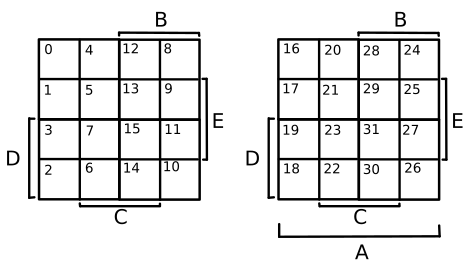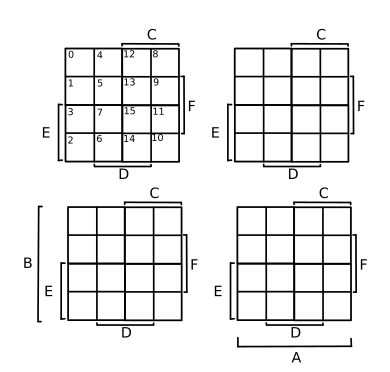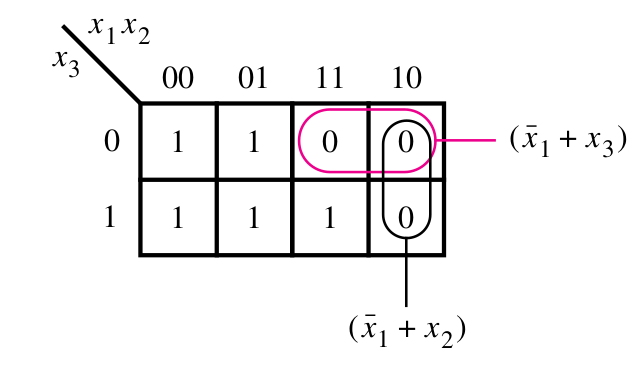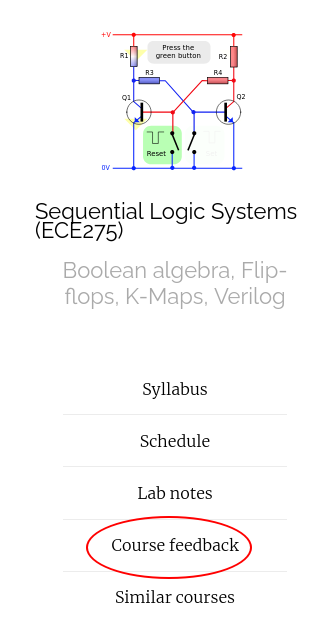## Karnaugh Maps terminology

• Literal : a single variable or its complement.
• Implicant: A product term that indicates for which $$f = 1$$
All minterms are implicants.
• Prime Implicant (PI): If an implicant cannot be "combined" into fewer literals.
• Essential Prime Implicant (EPI): A PI that is the only PI to cover some 1 on the K-map
• Cover : PI's that account for all $$f = 1$$
Example: $$f = \sum m(0, 1, 2, 3, 7) = \bx_1 + x_1 x_2 x_3$$

## Karnaugh Maps terminology

• Literal : a single variable or its complement.
• Implicant: A product term that indicates for which $$f = 1$$
All minterms are implicants.
• Prime Implicant (PI): If an implicant cannot be "combined" into fewer literals.
• Essential Prime Implicant (EPI): A PI that is the only PI to cover some 1 on the K-map.
• Cover : PI's that account for all $$f = 1$$.
• Cost : Number of gates and inputs excluding input complements.
Example: $$f = \sum m(0, 1, 2, 3, 7) = \bx_1 + x_1 x_2 x_3$$
$$\bar{x_1}$$ $$x_1$$
$$\bar{x_2}$$ $$x_2$$ $$\bar{y}$$
$$\bar{x_3}$$ 1 1 0 0
$$x_3$$ 1 1+1 1 0
• Literals are $$\bx_1, x_1, x_2, x_3$$
• Implicants are $$\bx_1$$, $$\bx_1 x_2 x_3, \dots$$, $$x_1 x_2 x_3$$
• PI's are $$\bx_1$$ and $$x_2 x_3$$
• EPI's are $$\bx_1$$ and $$x_2 x_3$$
• Cost is 9 = 6 inputs + 2 AND gate + 1 OR gate
Find the cost of the $$g = \overline{(x_1\bx_2 + x_3)}(\bx_4 + x_4)$$

Cost = 2 AND + 2 OR + 1 NOT + 9 inputs = 14

Find the cost of the $$f = (x_2 + x_3)(x_3 + x_4)(\bx_1 + \bx_2 + \bx_3 + \bx_4)$$

Cost = 3 OR + 1 AND + (8+3) inputs = 15

## K-map neighborhood property

\begin{align} f &= x_1 \bx_3 \bx_4 + x_2 \bx_3 \bx_4 + x_1 \bx_2 \bx_3 & \end{align}
$$\bar{x}_1$$ $$x_1$$
$$\bar{x}_2$$ $$x_2$$ $$\bar{x}_2$$
$$\bar{x}_3$$$$\bar{x}_4$$ 0 1 1 1
$$x_4$$ 0 0 0 1
$$x_3$$ 0 0 0 0
$$\bar{x}_4$$ 0 0 0 0

Adjacent cells of K-maps differ only by one variable

## Karnaugh Maps for 5-variable## Karnaugh Maps for 6-variable## K-Maps for POS minimization

$f(x_1, x_2, x_3) = \prod M(4, 5, 6)$
$f(x_1, x_2, x_3) = \prod M(4, 5, 6)$$f(x_1, x_2, x_3) = (\bx_1 + x_3)(\bx_1 + x_2)$

Cost = 9 = 6 inputs + 2 OR gate + 1 AND gate

$f(x_1, x_2, x_3) = \prod M(4, 5, 6)$ $\bar{f}(x_1, x_2, x_3) = \sum m(4, 5, 6)$
$$\bar{x}_1$$ $$x_1$$
$$\bar{x}_2$$ $$x_2$$ $$\bar{x}_2$$
$$\bar{x}_3$$ 0 0 1
1
$$x_3$$ 0 0 0 1
$\bar{f} = \color{red}{x_1 \bx_3} + \color{blue}{x_1 \bx_2 }$ $f = \color{red}{(\bx_1 + x_3)}\color{blue}{(\bx_1 + x_2)}$

## Minimization process

1. Draw K-map for $$f$$ and $$\bar{f}$$
2. Find cost for each. Choose the minimum cost implementation.

## Incompletely specified functions

$f(x_1, \dots, x_4) = \sum m(2, 4, 5, 6, 10) + D(12, 13, 14, 15).$
$f(x_1, \dots, x_4) = \sum m(2, 4, 5, 6, 10) + D(12, 13, 14, 15).$
$$\bar{x}_1$$ $$x_1$$
$$\bar{x}_2$$ $$x_2$$ $$\bar{x}_2$$
$$\bar{x}_3$$$$\bar{x}_4$$ 0 1 d 0
$$x_4$$ 0 1 d 0
$$x_3$$ 0 0 d 0
$$\bar{x}_4$$ 1 1 d 1
$f = \color{blue}{x_2 \bx_3} + \color{red}{x_3 \bx_4}$

Cost = 6 inputs + 2 AND + 1 OR = 7

$$\bar{x}_1$$ $$x_1$$
$$\bar{x}_2$$ $$x_2$$ $$\bar{x}_2$$
$$\bar{x}_3$$$$\bar{x}_4$$ 0 1 d 0
$$x_4$$ 0 1 d 0
$$x_3$$ 0 0 d 0
$$\bar{x}_4$$ 1 1 d 1
$\bar{f} = \color{blue}{\bx_2 \bx_3} + \color{red}{\bx_3 x_4}$ $f = \color{blue}{(x_2 + x_3)} \color{red}{(x_3 + \bx_4)}$

Cost = 6 inputs + 2 OR + 1 AND = 7

## Thanks, Questions, Feedback?

https://vikasdhiman.info/ECE275-Sequential-Logic/